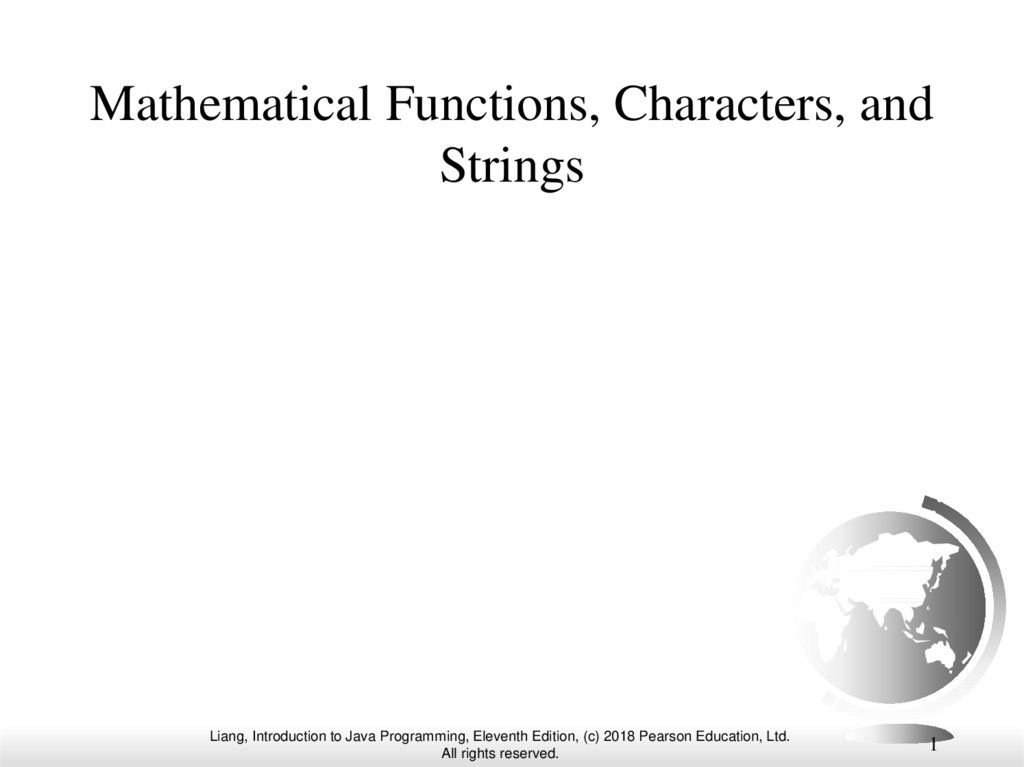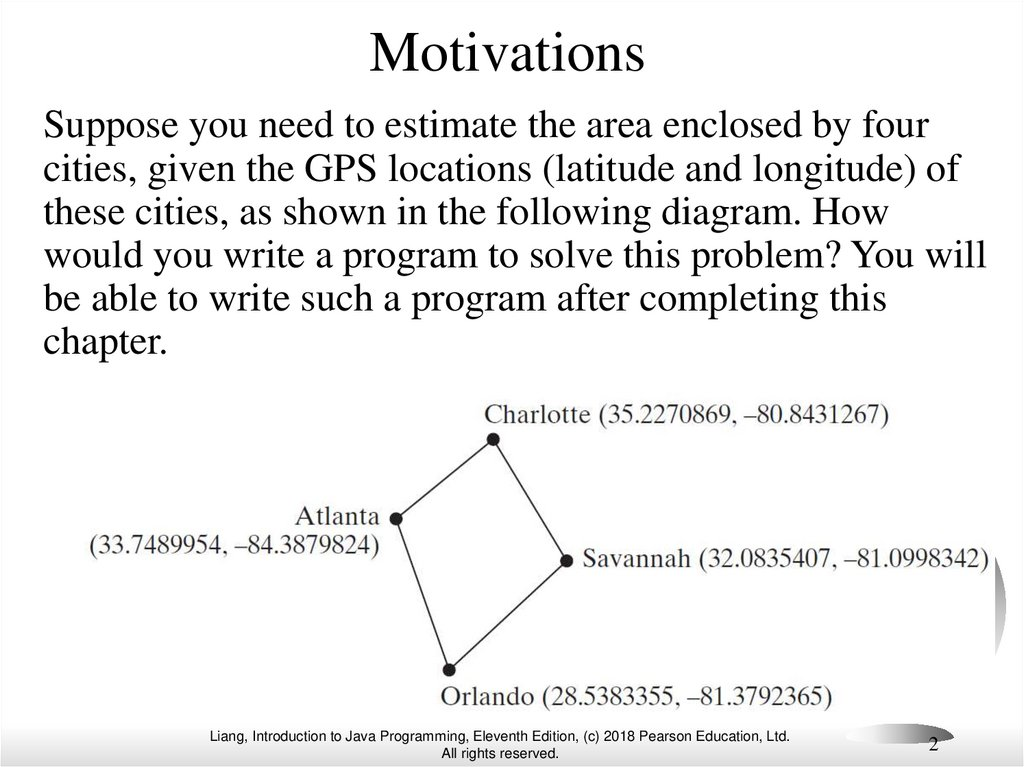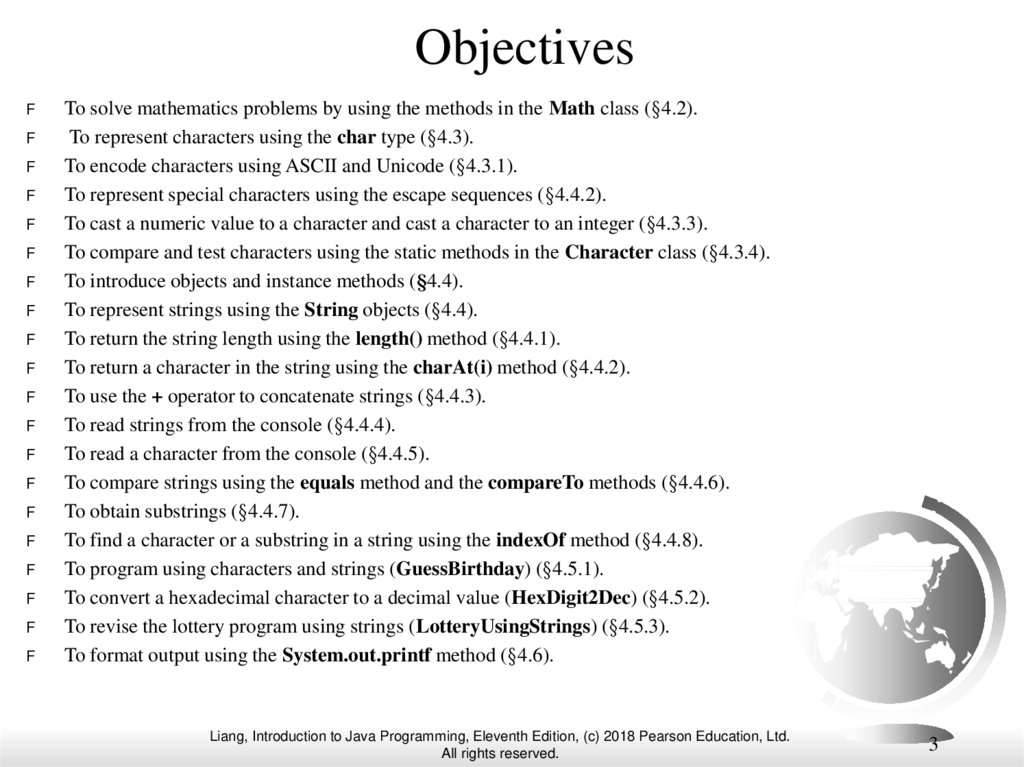Mathematical functions, characters, and strings. Introduction to Java Programming

1. Mathematical Functions, Characters, and Strings

Liang, Introduction to Java Programming, Eleventh Edition, (c) 2018 Pearson Education, Ltd.
1

2. Motivations

Suppose you need to estimate the area enclosed by four
cities, given the GPS locations (latitude and longitude) of
these cities, as shown in the following diagram. How
would you write a program to solve this problem? You will
be able to write such a program after completing this
chapter.
Liang, Introduction to Java Programming, Eleventh Edition, (c) 2018 Pearson Education, Ltd.
2

3. Objectives

F
F
F
F
F
F
F
F
F
F
F
F
F
F
F
F
F
F
F
F
To solve mathematics problems by using the methods in the Math class (§4.2).
To represent characters using the char type (§4.3).
To encode characters using ASCII and Unicode (§4.3.1).
To represent special characters using the escape sequences (§4.4.2).
To cast a numeric value to a character and cast a character to an integer (§4.3.3).
To compare and test characters using the static methods in the Character class (§4.3.4).
To introduce objects and instance methods (§4.4).
To represent strings using the String objects (§4.4).
To return the string length using the length() method (§4.4.1).
To return a character in the string using the charAt(i) method (§4.4.2).
To use the + operator to concatenate strings (§4.4.3).
To read strings from the console (§4.4.4).
To read a character from the console (§4.4.5).
To compare strings using the equals method and the compareTo methods (§4.4.6).
To obtain substrings (§4.4.7).
To find a character or a substring in a string using the indexOf method (§4.4.8).
To program using characters and strings (GuessBirthday) (§4.5.1).
To convert a hexadecimal character to a decimal value (HexDigit2Dec) (§4.5.2).
To revise the lottery program using strings (LotteryUsingStrings) (§4.5.3).
To format output using the System.out.printf method (§4.6).
Liang, Introduction to Java Programming, Eleventh Edition, (c) 2018 Pearson Education, Ltd.
3

4. Mathematical Functions

Java provides many useful methods in the Math
class for performing common mathematical
functions.
Liang, Introduction to Java Programming, Eleventh Edition, (c) 2018 Pearson Education, Ltd.
4

5. The Math Class

F
Class constants:
– PI
–E
F
Class methods:
– Trigonometric Methods
– Exponent Methods
– Rounding Methods
– min, max, abs, and random Methods
Liang, Introduction to Java Programming, Eleventh Edition, (c) 2018 Pearson Education, Ltd.
5

6. Trigonometric Methods

F
sin(double a)
F
cos(double a)
F
tan(double a)
F
acos(double a)
F
asin(double a)
F
atan(double a)
Examples:
Math.sin(0) returns 0.0
Math.sin(Math.PI / 6)
returns 0.5
Math.sin(Math.PI / 2)
returns 1.0
Math.cos(0) returns 1.0
Math.cos(Math.PI / 6)
returns 0.866
Math.cos(Math.PI / 2)
returns 0
Liang, Introduction to Java Programming, Eleventh Edition, (c) 2018 Pearson Education, Ltd.
6

7. Exponent Methods

F
exp(double a)
Returns e raised to the power of a.
Examples:
F
log(double a)
Returns the natural logarithm of a.
F
log10(double a)
Math.exp(1) returns 2.71
Math.log(2.71) returns 1.0
Math.pow(2, 3) returns 8.0
Math.pow(3, 2) returns 9.0
Math.pow(3.5, 2.5) returns
22.91765
Math.sqrt(4) returns 2.0
Math.sqrt(10.5) returns 3.24
Returns the 10-based logarithm of
a.
F
pow(double a, double b)
Returns a raised to the power of b.
F
sqrt(double a)
Returns the square root of a.
Liang, Introduction to Java Programming, Eleventh Edition, (c) 2018 Pearson Education, Ltd.
7

8. Rounding Methods

F
double ceil(double x)
x rounded up to its nearest integer. This integer is returned as a double
value.
F
double floor(double x)
x is rounded down to its nearest integer. This integer is returned as a
double value.
F
double rint(double x)
x is rounded to its nearest integer. If x is equally close to two integers,
the even one is returned as a double.
F
int round(float x)
Return (int)Math.floor(x+0.5).
F
long round(double x)
Return (long)Math.floor(x+0.5).
Liang, Introduction to Java Programming, Eleventh Edition, (c) 2018 Pearson Education, Ltd.
8

9. Rounding Methods Examples

Math.ceil(2.1) returns 3.0
Math.ceil(2.0) returns 2.0
Math.ceil(-2.0) returns –2.0
Math.ceil(-2.1) returns -2.0
Math.floor(2.1) returns 2.0
Math.floor(2.0) returns 2.0
Math.floor(-2.0) returns –2.0
Math.floor(-2.1) returns -3.0
Math.rint(2.1) returns 2.0
Math.rint(2.0) returns 2.0
Math.rint(-2.0) returns –2.0
Math.rint(-2.1) returns -2.0
Math.rint(2.5) returns 2.0
Math.rint(-2.5) returns -2.0
Math.round(2.6f) returns 3
Math.round(2.0) returns 2
Math.round(-2.0f) returns -2
Math.round(-2.6) returns -3
Liang, Introduction to Java Programming, Eleventh Edition, (c) 2018 Pearson Education, Ltd.
9

10. min, max, and abs

F
max(a, b)and min(a, b)
Returns the maximum or
minimum of two parameters.
F
abs(a)
Returns the absolute value of the
parameter.
F
random()
Returns a random double value
in the range [0.0, 1.0).
Examples:
Math.max(2, 3) returns 3
Math.max(2.5, 3) returns
3.0
Math.min(2.5, 3.6)
returns 2.5
Math.abs(-2) returns 2
Math.abs(-2.1) returns
2.1
Liang, Introduction to Java Programming, Eleventh Edition, (c) 2018 Pearson Education, Ltd.
10

11. The random Method

Generates a random double value greater than or equal to 0.0 and less
than 1.0 (0 <= Math.random() < 1.0).
Examples:
(int)(Math.random() * 10)
Returns a random integer
between 0 and 9.
50 + (int)(Math.random() * 50)
Returns a random integer
between 50 and 99.
In general,
a + Math.random() * b
Returns a random number between
a and a + b, excluding a + b.
Liang, Introduction to Java Programming, Eleventh Edition, (c) 2018 Pearson Education, Ltd.
11

12. Case Study: Computing Angles of a Triangle

x2, y2
c
A = acos((a * a - b * b - c * c) / (-2 * b * c))
B = acos((b * b - a * a - c * c) / (-2 * a * c))
C = acos((c * c - b * b - a * a) / (-2 * a * b))
a
B
C
x3, y3
A
b
x1, y1
Write a program that prompts the user to enter the
x- and y-coordinates of the three corner points in a
triangle and then displays the triangle’s angles.
IMPORTANT NOTE: If you cannot run the buttons, see
https://liveexample.pearsoncmg.com/slide/javaslidenote.doc.
ComputeAngles
Liang, Introduction to Java Programming, Eleventh Edition, (c) 2018 Pearson Education, Ltd.
Run
12

13. Character Data Type

char letter = 'A'; (ASCII)
char numChar = '4'; (ASCII)
char letter = '\u0041'; (Unicode)
char numChar = '\u0034'; (Unicode)
NOTE: The increment and decrement operators can also be used
on char variables to get the next or preceding Unicode character.
For example, the following statements display character b.
char ch = 'a';
System.out.println(++ch);
Liang, Introduction to Java Programming, Eleventh Edition, (c) 2018 Pearson Education, Ltd.
13

14. Unicode Format

Java characters use Unicode, a 16-bit encoding scheme
established by the Unicode Consortium to support the
interchange, processing, and display of written texts in the
world’s diverse languages. Unicode takes two bytes,
preceded by \u, expressed in four hexadecimal numbers
that run from '\u0000' to '\uFFFF'. So, Unicode can
represent 65535 + 1 characters.
Unicode \u03b1 \u03b2 \u03b3 for three Greek
letters
Liang, Introduction to Java Programming, Eleventh Edition, (c) 2018 Pearson Education, Ltd.
14

15. ASCII Code for Commonly Used Characters

Characters
Code Value in Decimal
Unicode Value
'0' to '9'
'A' to 'Z'
'a' to 'z'
48 to 57
65 to 90
97 to 122
\u0030 to \u0039
\u0041 to \u005A
\u0061 to \u007A
Liang, Introduction to Java Programming, Eleventh Edition, (c) 2018 Pearson Education, Ltd.
15

16. Escape Sequences for Special Characters

Liang, Introduction to Java Programming, Eleventh Edition, (c) 2018 Pearson Education, Ltd.
16

17. Appendix B: ASCII Character Set

ASCII Character Set is a subset of the Unicode from \u0000 to \u007f
Liang, Introduction to Java Programming, Eleventh Edition, (c) 2018 Pearson Education, Ltd.
17

18. ASCII Character Set, cont.

ASCII Character Set is a subset of the Unicode from \u0000 to \u007f
Liang, Introduction to Java Programming, Eleventh Edition, (c) 2018 Pearson Education, Ltd.
18

19. Casting between char and Numeric Types

int i = 'a'; // Same as int i = (int)'a';
char c = 97; // Same as char c = (char)97;
Liang, Introduction to Java Programming, Eleventh Edition, (c) 2018 Pearson Education, Ltd.
19

20. Comparing and Testing Characters

if (ch >= 'A' && ch <= 'Z')
System.out.println(ch + " is an uppercase letter");
else if (ch >= 'a' && ch <= 'z')
System.out.println(ch + " is a lowercase letter");
else if (ch >= '0' && ch <= '9')
System.out.println(ch + " is a numeric character");
Liang, Introduction to Java Programming, Eleventh Edition, (c) 2018 Pearson Education, Ltd.
20

21. Methods in the Character Class

Method
Description
isDigit(ch)
Returns true if the specified character is a digit.
isLetter(ch)
Returns true if the specified character is a letter.
isLetterOfDigit(ch)
Returns true if the specified character is a letter or digit.
isLowerCase(ch)
Returns true if the specified character is a lowercase letter.
isUpperCase(ch)
Returns true if the specified character is an uppercase letter.
toLowerCase(ch)
Returns the lowercase of the specified character.
toUpperCase(ch)
Returns the uppercase of the specified character.
Liang, Introduction to Java Programming, Eleventh Edition, (c) 2018 Pearson Education, Ltd.
21

22. The String Type

The char type only represents one character. To represent a string
of characters, use the data type called String. For example,
String message = "Welcome to Java";
String is actually a predefined class in the Java library just like the
System class and Scanner class. The String type is not a primitive
type. It is known as a reference type. Any Java class can be used
as a reference type for a variable. Reference data types will be
thoroughly discussed in Chapter 9, “Objects and Classes.” For the
time being, you just need to know how to declare a String
variable, how to assign a string to the variable, how to concatenate
strings, and to perform simple operations for strings.
Liang, Introduction to Java Programming, Eleventh Edition, (c) 2018 Pearson Education, Ltd.
22

23. Simple Methods for String Objects

Method
Description
length()
charAt(index)
Returns the number of characters in this string.
concat(s1)
toUpperCase()
Returns a new string that concatenates this string with string s1.
toLowerCase()
trim()
Returns a new string with all letters in lowercase.
Returns the character at the specified index from this string.
Returns a new string with all letters in uppercase.
Returns a new string with whitespace characters trimmed on both sides.
Liang, Introduction to Java Programming, Eleventh Edition, (c) 2018 Pearson Education, Ltd.
23

24. Simple Methods for String Objects

Strings are objects in Java. The methods in the preceding
table can only be invoked from a specific string instance.
For this reason, these methods are called instance methods.
A non-instance method is called a static method. A static
method can be invoked without using an object. All the
methods defined in the Math class are static methods. They
are not tied to a specific object instance. The syntax to
invoke an instance method is
referenceVariable.methodName(arguments).
Liang, Introduction to Java Programming, Eleventh Edition, (c) 2018 Pearson Education, Ltd.
24

25. Getting String Length

String message = "Welcome to Java";
System.out.println("The length of " + message + " is "
+ message.length());
Liang, Introduction to Java Programming, Eleventh Edition, (c) 2018 Pearson Education, Ltd.
25

26. Getting Characters from a String

String message = "Welcome to Java";
System.out.println("The first character in message is "
+ message.charAt(0));
Liang, Introduction to Java Programming, Eleventh Edition, (c) 2018 Pearson Education, Ltd.
26

27. Converting Strings

"Welcome".toLowerCase() returns a new string, welcome.
"Welcome".toUpperCase() returns a new string,
WELCOME.
" Welcome ".trim() returns a new string, Welcome.
Liang, Introduction to Java Programming, Eleventh Edition, (c) 2018 Pearson Education, Ltd.
27

28. String Concatenation

String s3 = s1.concat(s2); or String s3 = s1 + s2;
// Three strings are concatenated
String message = "Welcome " + "to " + "Java";
// String Chapter is concatenated with number 2
String s = "Chapter" + 2; // s becomes Chapter2
// String Supplement is concatenated with character B
String s1 = "Supplement" + 'B'; // s1 becomes SupplementB
Liang, Introduction to Java Programming, Eleventh Edition, (c) 2018 Pearson Education, Ltd.
28

29. Reading a String from the Console

Scanner input = new Scanner(System.in);
System.out.print("Enter three words separated by spaces: ");
String s1 = input.next();
String s2 = input.next();
String s3 = input.next();
System.out.println("s1 is " + s1);
System.out.println("s2 is " + s2);
System.out.println("s3 is " + s3);
Liang, Introduction to Java Programming, Eleventh Edition, (c) 2018 Pearson Education, Ltd.
29

30. Reading a Character from the Console

Scanner input = new Scanner(System.in);
System.out.print("Enter a character: ");
String s = input.nextLine();
char ch = s.charAt(0);
System.out.println("The character entered is " + ch);
Liang, Introduction to Java Programming, Eleventh Edition, (c) 2018 Pearson Education, Ltd.
30

31. Comparing Strings

Method
Description
equals(s1)
Returns true if this string is equal to string s1.
equalsIgnoreCase(s1)
compareTo(s1)
Returns true if this string is equal to string s1; it is case insensitive.
Returns an integer greater than 0, equal to 0, or less than 0 to indicate whether
this string is greater than, equal to, or less than s1.
compareToIgnoreCase(s1) Same as compareTo except that the comparison is case insensitive.
Returns true if this string starts with the specified prefix.
startsWith(prefix)
Returns true if this string ends with the specified suffix.
endsWith(suffix)
OrderTwoCities
Liang, Introduction to Java Programming, Eleventh Edition, (c) 2018 Pearson Education, Ltd.
Run
31

32. Obtaining Substrings

Method
Description
substring(beginIndex)
Returns this string’s substring that begins with the character at the specified
beginIndex and extends to the end of the string, as shown in Figure 4.2.
substring(beginIndex,
endIndex)
Returns this string’s substring that begins at the specified beginIndex and
extends to the character at index endIndex – 1, as shown in Figure 9.6.
Note that the character at endIndex is not part of the substring.
Liang, Introduction to Java Programming, Eleventh Edition, (c) 2018 Pearson Education, Ltd.
32

33. Finding a Character or a Substring in a String

Method
Description
indexOf(ch)
Returns the index of the first occurrence of ch in the string. Returns -1 if not
matched.
indexOf(ch, fromIndex)
Returns the index of the first occurrence of ch after fromIndex in the string.
Returns -1 if not matched.
indexOf(s)
Returns the index of the first occurrence of string s in this string. Returns -1 if
not matched.
indexOf(s, fromIndex)
Returns the index of the first occurrence of string s in this string after
fromIndex. Returns -1 if not matched.
lastIndexOf(ch)
Returns the index of the last occurrence of ch in the string. Returns -1 if not
matched.
lastIndexOf(ch,
fromIndex)
lastIndexOf(s)
Returns the index of the last occurrence of ch before fromIndex in this
string. Returns -1 if not matched.
lastIndexOf(s,
fromIndex)
Returns the index of the last occurrence of string s before fromIndex.
Returns -1 if not matched.
Returns the index of the last occurrence of string s. Returns -1 if not matched.
Liang, Introduction to Java Programming, Eleventh Edition, (c) 2018 Pearson Education, Ltd.
33

34. Finding a Character or a Substring in a String

int k = s.indexOf(' ');
String firstName = s.substring(0, k);
String lastName = s.substring(k + 1);
Liang, Introduction to Java Programming, Eleventh Edition, (c) 2018 Pearson Education, Ltd.
34

35. Conversion between Strings and Numbers

int intValue = Integer.parseInt(intString);
double doubleValue = Double.parseDouble(doubleString);
String s = number + "";
Liang, Introduction to Java Programming, Eleventh Edition, (c) 2018 Pearson Education, Ltd.
35

36. Problem: Guessing Birthday

The program can guess your birth date. Run
to see how it works.
GuessBirthday
Liang, Introduction to Java Programming, Eleventh Edition, (c) 2018 Pearson Education, Ltd.
Run
36

37. Mathematics Basis for the Game

19 is 10011 in binary. 7 is 111 in binary. 23 is 11101 in binary
10000
10
+
1
10011
19
00110
10
+
1
00111
7
10000
1000
100
+
1
11101
23
Liang, Introduction to Java Programming, Eleventh Edition, (c) 2018 Pearson Education, Ltd.
37

38. Case Study: Converting a Hexadecimal Digit to a Decimal Value

Write a program that converts a hexadecimal digit
into a decimal value.
HexDigit2Dec
Liang, Introduction to Java Programming, Eleventh Edition, (c) 2018 Pearson Education, Ltd.
Run
38

39. Case Study: Revising the Lottery Program Using Strings

A problem can be solved using many different approaches.
This section rewrites the lottery program in Listing 3.7
using strings. Using strings simplifies this program.
LotteryUsingStrings
Liang, Introduction to Java Programming, Eleventh Edition, (c) 2018 Pearson Education, Ltd.
Run
39

40. Formatting Output

Use the printf statement.
System.out.printf(format, items);
Where format is a string that may consist of substrings and
format specifiers. A format specifier specifies how an item
should be displayed. An item may be a numeric value,
character, boolean value, or a string. Each specifier begins
with a percent sign.
Liang, Introduction to Java Programming, Eleventh Edition, (c) 2018 Pearson Education, Ltd.
40

41. Frequently-Used Specifiers

Specifier Output
Example
%b
a boolean value
true or false
%c
a character
'a'
%d
a decimal integer
200
%f
a floating-point number
45.460000
%e
a number in standard scientific notation
4.556000e+01
%s
a string
"Java is cool"
int count = 5;
items
double amount = 45.56;
System.out.printf("count is %d and amount is %f", count, amount);
display
count is 5 and amount is 45.560000
Liang, Introduction to Java Programming, Eleventh Edition, (c) 2018 Pearson Education, Ltd.